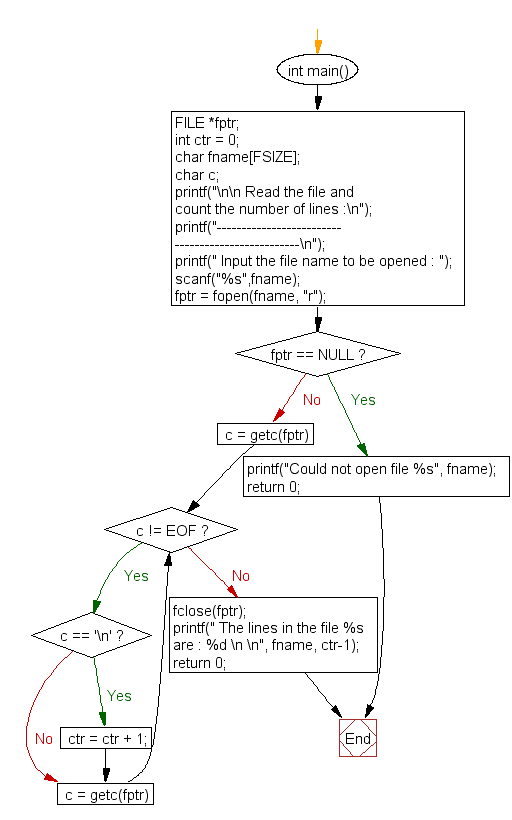﻿ C Program: Read the file and count the number of lines - w3resource# C Exercises: Read the file and count the number of lines

## C File Handling : Exercise-5 with Solution

Write a program in C to Find the Number of Lines in a Text File.

Sample Solution:

C Code:

``````#include <stdio.h>

#define FSIZE 100

int main()
{
FILE *fptr;
int ctr = 0;
char fname[FSIZE];
char c;
printf("\n\n Read the file and count the number of lines :\n");
printf("--------------------------------------------------\n");
printf(" Input the file name to be opened : ");
scanf("%s",fname);

fptr = fopen(fname, "r");
if (fptr == NULL)
{
printf("Could not open file %s", fname);
return 0;
}
// Extract characters from file and store in character c
for (c = getc(fptr); c != EOF; c = getc(fptr))
if (c == '\n') // Increment count if this character is newline
ctr = ctr + 1;
fclose(fptr);
printf(" The lines in the file %s are : %d \n \n", fname, ctr-1);
return 0;
}
```
```

Sample Output:

``` Read the file and count the number of lines :
--------------------------------------------------
Input the file name to be opened : test.txt
The lines in the file test.txt are : 4
```

Flowchart:C Programming Code Editor:

Have another way to solve this solution? Contribute your code (and comments) through Disqus.

What is the difficulty level of this exercise?

Test your Programming skills with w3resource's quiz.

﻿

## C Programming: Tips of the Day

What is the size of an enum in c?

An enum is only guaranteed to be large enough to hold int values. The compiler is free to choose the actual type used based on the enumeration constants defined so it can choose a smaller type if it can represent the values you define. If you need enumeration constants that don't fit into an int you will need to use compiler-specific extensions to do so.

Ref : https://bit.ly/3ypg4k8